Next: Multi-Slit Diffraction Up: Wave Optics Previous: One-Dimensional Fourier Optics

# Single-Slit Diffraction

Suppose that the opaque screen contains a single slit of finite width. In fact, let the slit in question be of width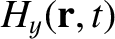, and extend from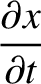to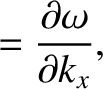. The associated aperture function is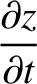(1046)

It follows from Equation (1044) that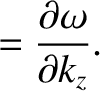(1047)

where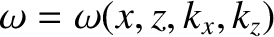. Finally, assuming, for the sake of simplicity, that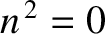, which is most likely to be the case when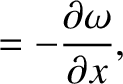, the diffraction pattern appearing on the projection screen is specified by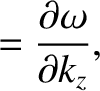(1048)

According to L'Hopital's rule,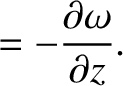. Furthermore, it is easily demonstrated that the zeros of the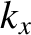function occur at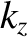, where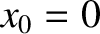is a non-zero integer.Figure 71 shows a typical single-slit diffraction pattern calculated for a case in which the slit width greatly exceeds the wavelength of the light. The pattern consists of a dominant central maximum, flanked by subsidiary maxima of fairly negligible amplitude. The situation is shown schematically in Figure 72. When the incident plane wave, whose direction of propagation subtends an anglewith the-axis, passes through the slit it is effectively transformed into a divergent beam of light (the beam corresponds to the central peak in Figure 71) that is centered on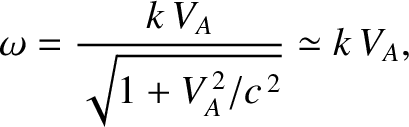. The angle of divergence of the beam, which is obtained from the first zero of the single-slit diffraction function (1048), is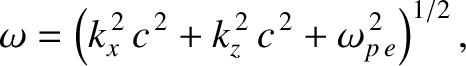(1049)

that is, the beam effectively extends from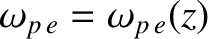to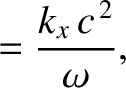. Thus, if the slit width,, is very much greater than the wavelength,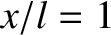, of the light then the beam divergence is negligible, and the beam is, thus, governed by the laws of geometric optics (according to which there is no beam divergence). On the other hand, if the slit width is comparable with the wavelength of the light then the beam divergence is significant, and the behavior of the beam is, consequently, very different than that predicted by the laws of geometric optics.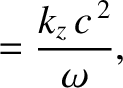The diffraction of light is an important physical phenomenon because it sets a limit on the angular resolution of optical instruments. For instance, consider a telescope whose objective lens is of diameter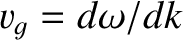. When a plane wave from a distant light source of negligible angular extent (e.g., a star) enter the lens it is diffracted, and forms a divergent beam of angular width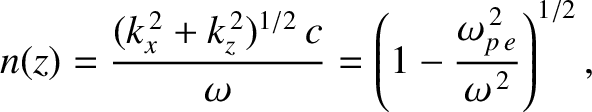. Thus, instead of being a point, the resulting image of the star is a disk of finite angular width. (See Section 11.9.) Suppose that two stars are an angular distance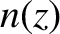apart in the sky. As we have just seen, when viewed through the telescope, each star appears as a disk of angular extent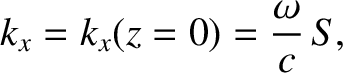. If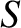then the two stars appear as separate disks. On the other hand, if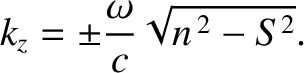then the two disks merge to form a single disk, and it becomes impossible to tell that there are, in fact, two stars. It follows that the maximum angular resolution of a telescope whose objective lens is of diameteris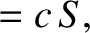(1050)

This result is called the Rayleigh criterion. (See Section 11.9 for a more accurate version of this criterion.) It can be seen that the angular resolution of the telescope increases (i.e.,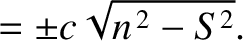decreases) as the diameter of its objective lens increases.Next: Multi-Slit Diffraction Up: Wave Optics Previous: One-Dimensional Fourier Optics
Richard Fitzpatrick 2013-04-08